# OpenGL ES for Android（投光物）

## 简介

### 定向光``````  struct Light {
// vec3 position; // 使用定向光就不再需要位置了
vec3 direction;

vec3 ambient;
vec3 diffuse;
vec3 specular;
};
...
void main()
{
vec3 lightDir = normalize(-(aLightMatrix *light.direction));
...
}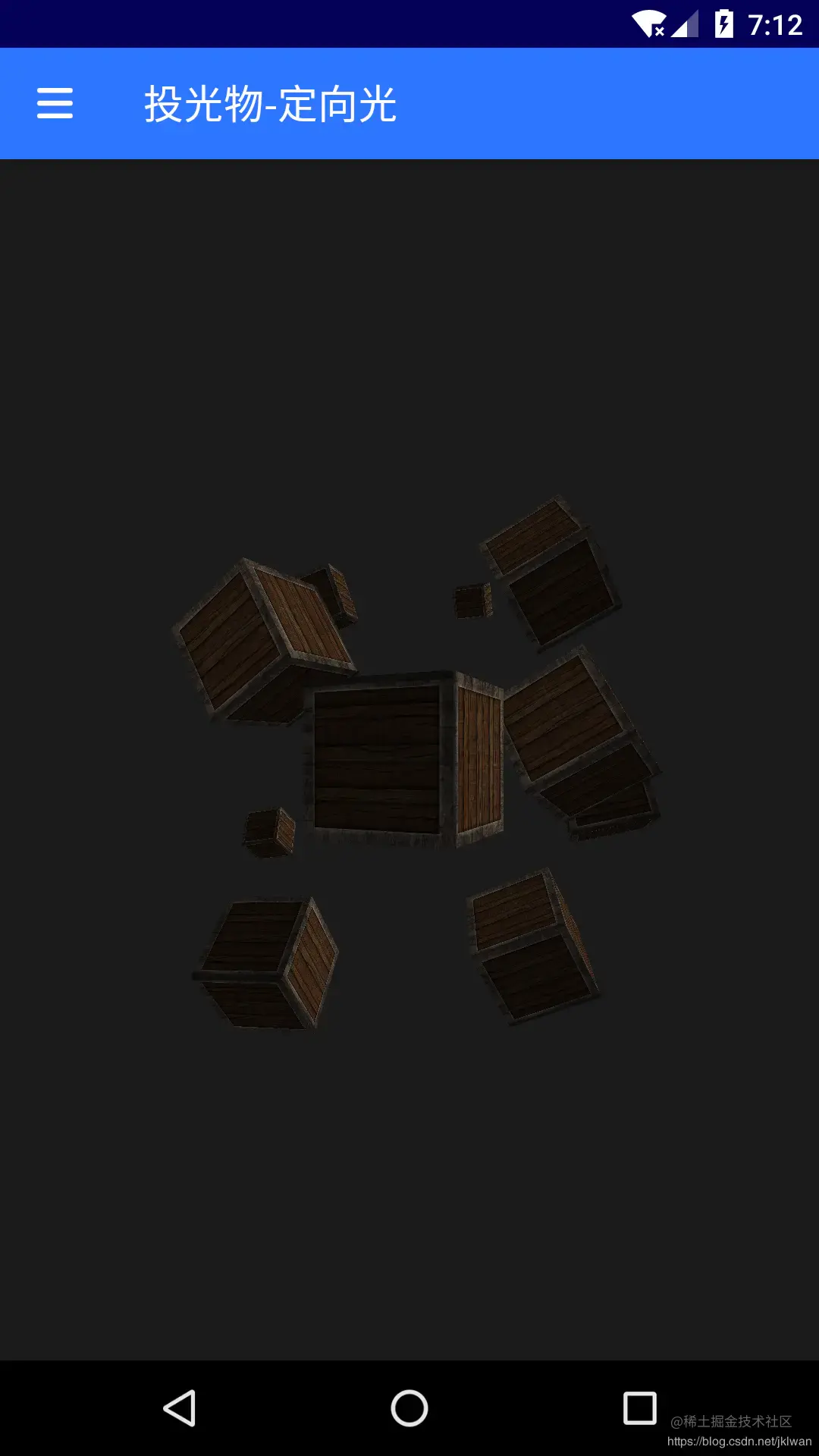### 点光源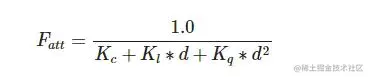• 常数项通常保持为1.0，它的主要作用是保证分母永远不会比1小，否则的话在某些距离上它反而会增加强度，这肯定不是我们想要的效果。
• 一次项会与距离值相乘，以线性的方式减少强度。
• 二次项会与距离的平方相乘，让光源以二次递减的方式减少强度。* 二次项在距离比较小的时候影响会比一次项小很多，但当距离值比较大的时候它就会比一次项更大了。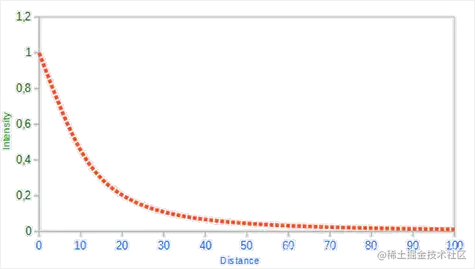``````  ……
struct Light {

……
float constant;
float linear;
};
……
void main() {
……
// 衰减
float distance = length(light.position - fragPos);
float attenuation = 1.0 / (light.constant + light.linear * distance +
ambient *= attenuation;
diffuse *= attenuation;
specular *= attenuation;
// 结果
vec3 result = ambient + diffuse + specular;

gl_FragColor = vec4(result, 1.0);
}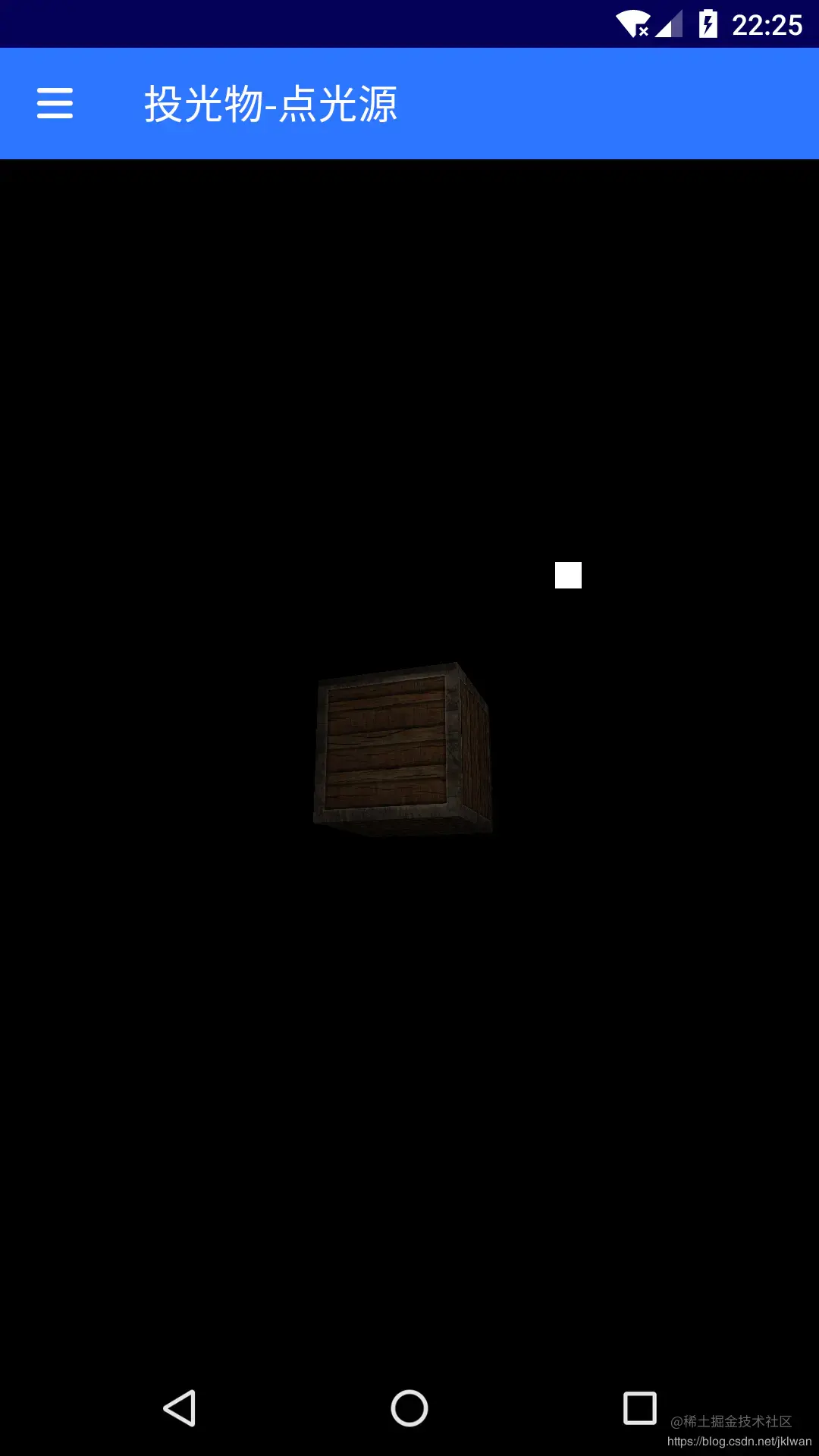### 聚光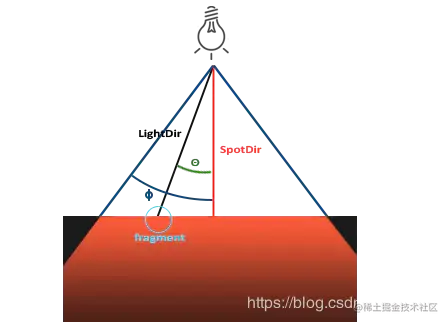`````` // 定义光源结构体
struct Light {
vec3 position;
vec3 direction;
float cutOff;
};

``````  ……
void main() {
vec3 lightDir = normalize(light.position - fragPos);

// 检查当前点与光源的连线是否在聚光灯内
float theta = dot(lightDir, normalize(-(aLightMatrix *
light.direction)));

if(theta > light.cutOff){
// 被照射到
……
} else {
// 没有被照射到
gl_FragColor = vec4(light.ambient *
texture2D(material.diffuse, TextCoord).rgb, 1.0);
}

}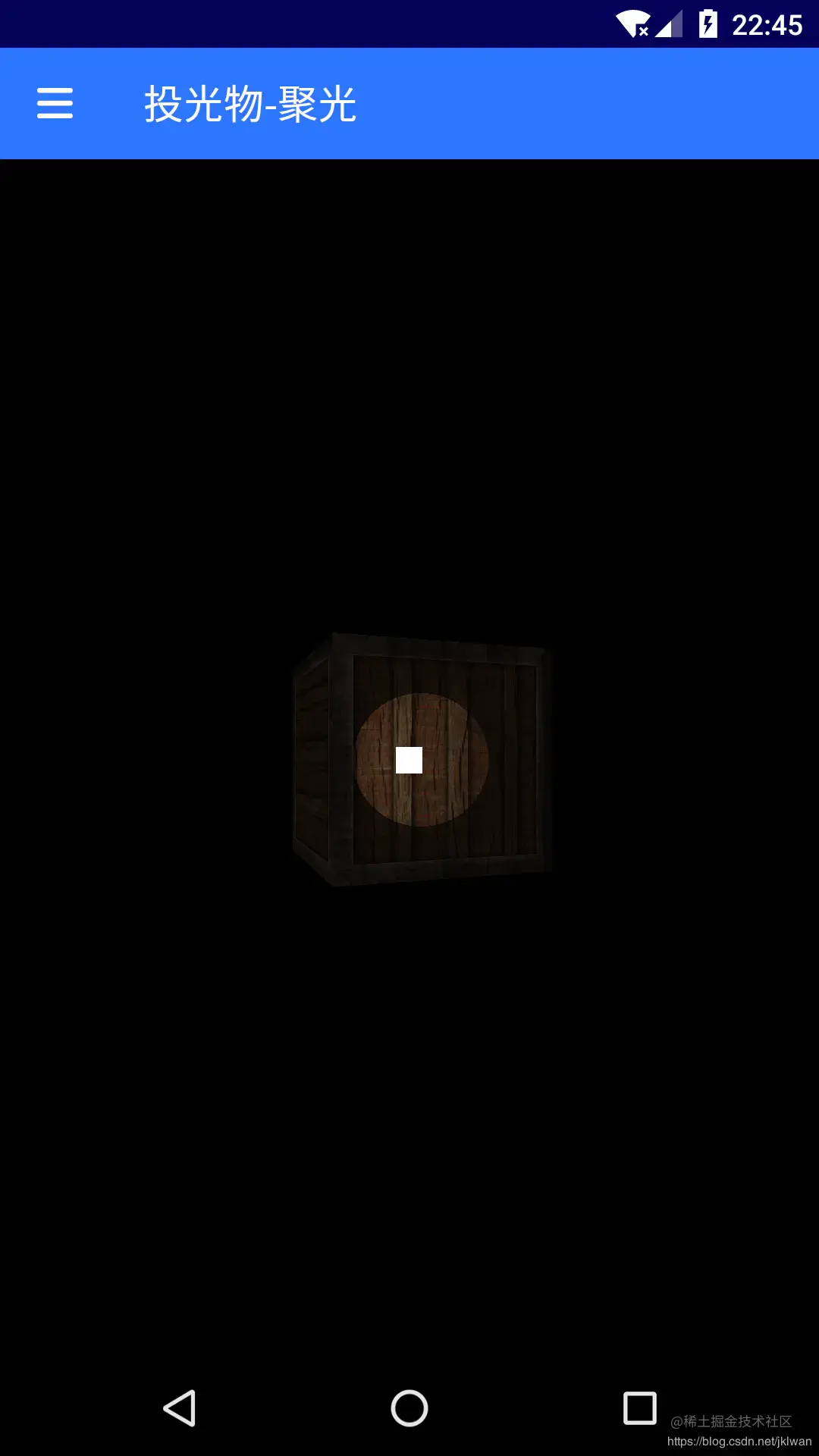``````  float theta = dot(lightDir, normalize(-light.direction));
float epsilon = light.cutOff - light.outerCutOff;
float intensity = clamp((theta - light.outerCutOff) / epsilon, 0.0,
1.0);
……
diffuse  *= intensity;
specular *= intensity;
……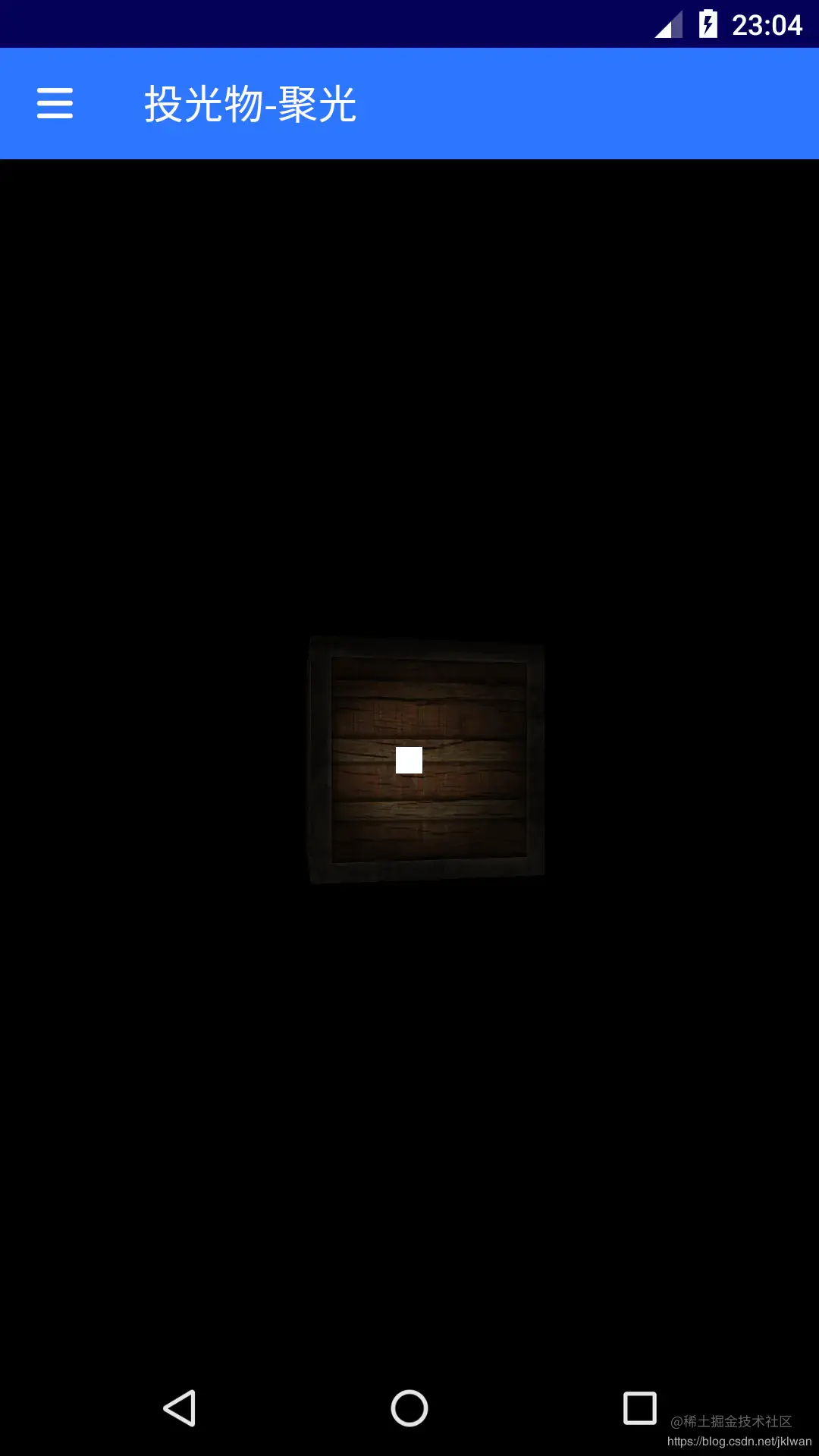Android

Android